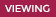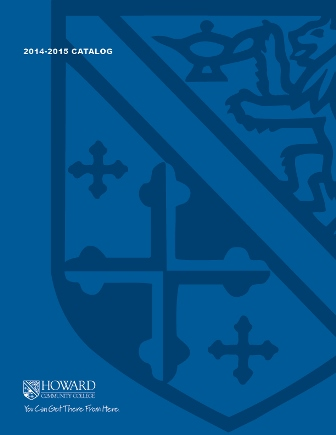2021-2022 College CatalogMATH 143 Precalculus I

This course can be taken as a single semester course or as the first part of a two semester sequence to prepare for calculus. Topics include absolute values, polynomial, rational, exponential, and logarithmic functions. Problems will be solved through analytical, numerical and graphical approaches with an emphasis on application problems. Graphing calculators and mathematical software will be employed throughout the course. All sections require the use of the interactive computer program that comes with the software code.

3

Prerequisite

MATH 070, MATH 073, or appropriate score on mathematics placement test

3 hours weekly

Course Objectives

1. Interpret and communicate mathematical concepts related to precalculus topics using appropriate symbols, notation, and vocabulary.
2. Use various representations (graphs, tables, and equations) of absolute value, polynomial, power, rational, exponential, and logarithmic functions to describe the characteristics of the functions and solve problems.
3. Identify and graph shifts, reflections, and transformations of functions.
4. Find inverses of functions algebraically as well as graphically.
5. Apply classic theorems to find the zeros of polynomial equations.
6. Use properties of logarithms and exponents to rewrite and evaluate logarithmic and exponential expressions.
7. Use mathematical models to solve application models.
8. Solve application problems modeled by absolute value, quadratic, polynomial, power, and root functions using both analytical and graphical strategies.
9. Analyze, evaluate, justify, and interpret the reasonableness of solutions.

Course Objectives

1. Interpret and communicate mathematical concepts related to precalculus topics using appropriate symbols, notation, and vocabulary.

Learning Activity Artifact

• Other (please fill out box below)
• Quizzes

Procedure for Assessing Student Learning

• Quantitative Reasoning Rubric

Quantitative Reasoning

• QR1
2. Use various representations (graphs, tables, and equations) of absolute value, polynomial, power, rational, exponential, and logarithmic functions to describe the characteristics of the functions and solve problems.

Learning Activity Artifact

• Other (please fill out box below)
• Quizzes

Procedure for Assessing Student Learning

• Quantitative Reasoning Rubric

Quantitative Reasoning

• QR2
3. Identify and graph shifts, reflections, and transformations of functions.

This objective is a course Goal Only

Learning Activity Artifact

• Other (please fill out box below)
• Exams

Procedure for Assessing Student Learning

• Quantitative Reasoning Rubric
4. Find inverses of functions algebraically as well as graphically.

This objective is a course Goal Only

Learning Activity Artifact

• Other (please fill out box below)
• Exams

Procedure for Assessing Student Learning

• Quantitative Reasoning Rubric
5. Apply classic theorems to find the zeros of polynomial equations.

This objective is a course Goal Only

Learning Activity Artifact

• Other (please fill out box below)
• Exams

Procedure for Assessing Student Learning

• Quantitative Reasoning Rubric
6. Use properties of logarithms and exponents to rewrite and evaluate logarithmic and exponential expressions.

This objective is a course Goal Only

Learning Activity Artifact

• Other (please fill out box below)
• Exams

Procedure for Assessing Student Learning

• Quantitative Reasoning Rubric
7. Use mathematical models to solve application models.

Learning Activity Artifact

• Other (please fill out box below)
• Quizzes

Procedure for Assessing Student Learning

• Quantitative Reasoning Rubric

Quantitative Reasoning

• QR4
8. Solve application problems modeled by absolute value, quadratic, polynomial, power, and root functions using both analytical and graphical strategies.

Learning Activity Artifact

• Other (please fill out box below)
• Quizzes

Procedure for Assessing Student Learning

• Quantitative Reasoning Rubric

Quantitative Reasoning

• QR3
9. Analyze, evaluate, justify, and interpret the reasonableness of solutions.

This objective is a course Goal Only

Learning Activity Artifact

• Other (please fill out box below)
• Exams

Procedure for Assessing Student Learning

• Quantitative Reasoning Rubric